"Lena" Image with Different Types of Distortions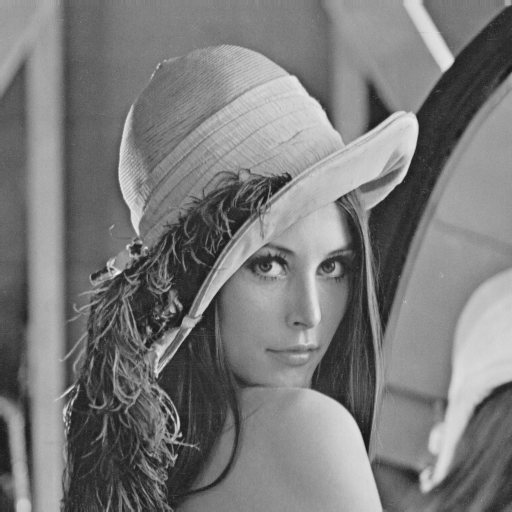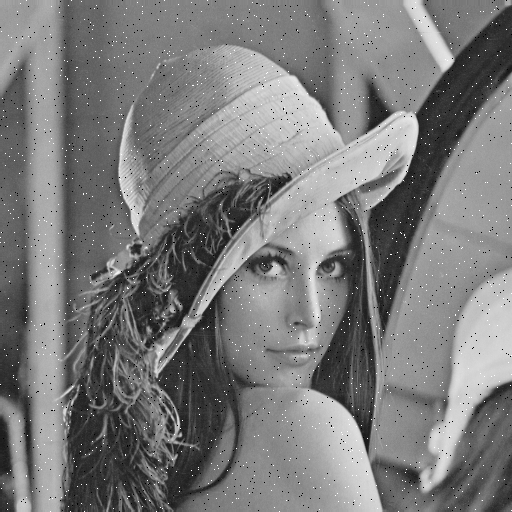(a) Original "Lena" image, 512 by 512, 8bits/pixel MSE = 0, Q = 1 (b) Impulsive Salt-Pepper Noise MSE = 225, Q = 0.6494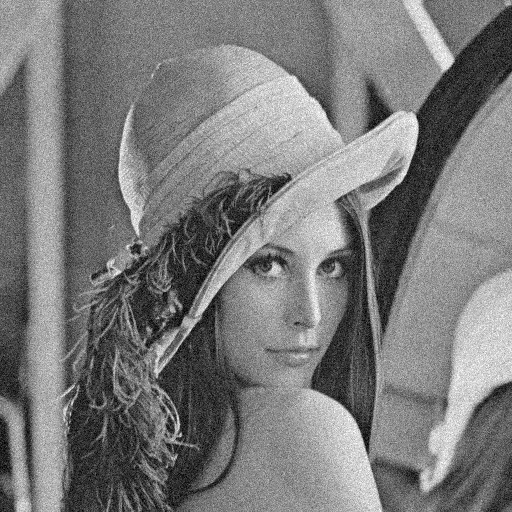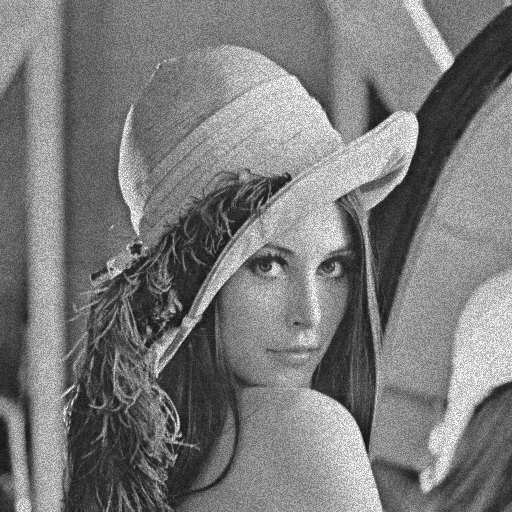(c) Additive Gaussian Noise MSE = 225, Q = 0.3891 (d) Multiplicative Speckle Noise MSE = 225, Q = 0.4408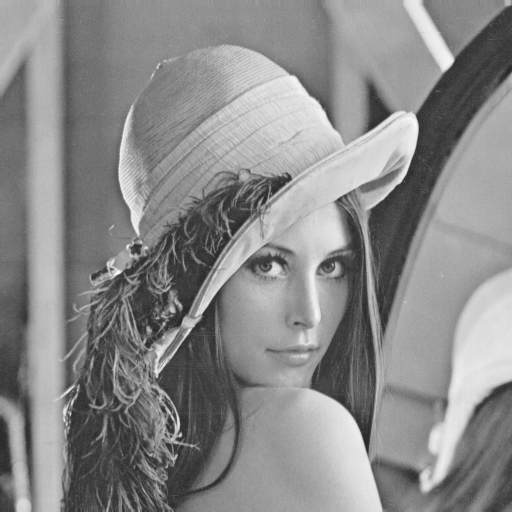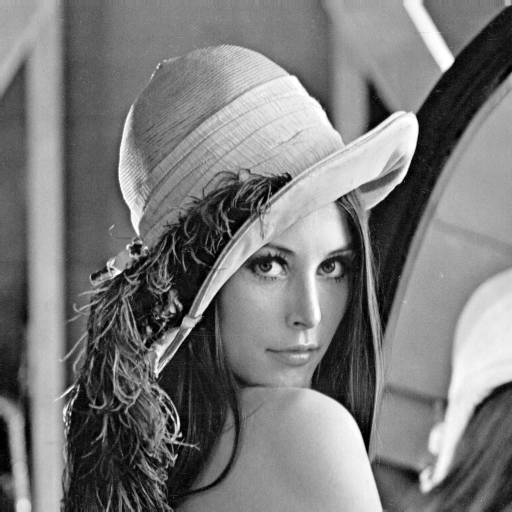(e) Mean Shift MSE = 225, Q = 0.9894 (f) Contrast Stretching MSE = 225, Q = 0.9372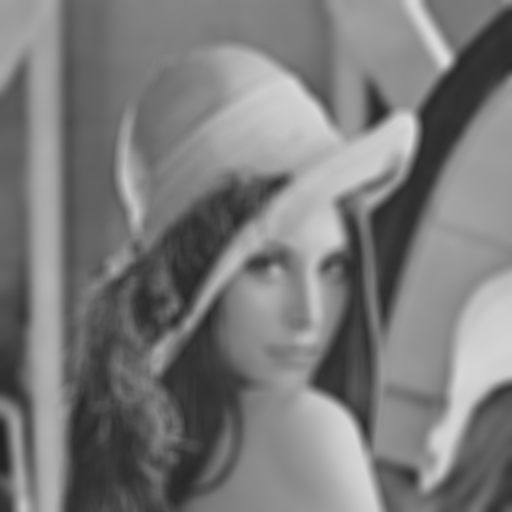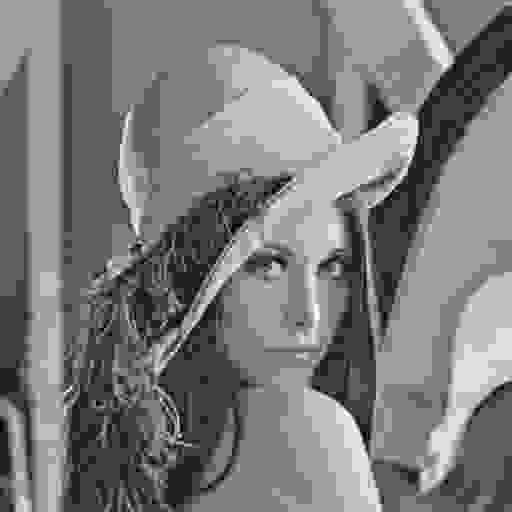(g) Blurring MSE = 225, Q = 0.3461 (h) JPEG Compression MSE = 215, Q = 0.2876

MSE: mean squared error; Q: the proposed universal image quality index

Note: All distorted images have nearly identical MSE, but with drastically different visual quality.

Back to Zhou Wang's Research Work

Back to Zhou Wang's Homepage

Back to LIVE

Last updated Feb. 27, 2001